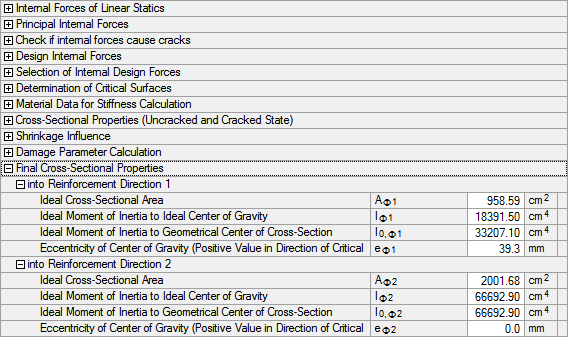# RF-CONCRETE Surfaces – Online Manual Version 5

Online manuals, introductory examples, tutorials, and other documentation.

# 2.7.10.8 Final cross-section properties

#### Final cross-section properties

The curvature for both crack states c (cracked/uncracked) is calculated as follows:

• uncracked state:

• cracked state:

The strain for both crack states is determined as follows:

• uncracked state:

• cracked state:

Thus, it is possible to determine the mean strain.

The mean curvature is determined as follows:

With the mean curvature and the longitudinal strain, you can calculate the final cross-section properties while taking account of shrinkage, creep, and tension stiffening.

Ideal cross-section area

Ideal moment of inertia to the ideal center of the cross-section

Eccentricity of centroid

Ideal moment of inertia to the geometric center of the cross-section

Poisson's ratio is determined as follows:Figure 2.124 Final cross-section properties